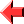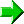﻿ TLWNN Tutorial Lesson 5: Input and Strings#Lesson 5## Receiving Input

The second of the library stacks (just below the one containing procedures for arithmetic and conditionality) is a stack comprising two procedures to do with input.

Let's look at the lower one first.  It simply reads one character from the standard input.  The Unicode value of this character is then pushed to the main stack.  (I talked of ASCII before; in fact TLWNN works in Unicode.  But that doesn't matter, as the way it's done doesn't affect how to output an ASCII character, which is what that was talking about.)

``````Example nine
Read a single character and then write it out
@!<!!
``````

## Strings and Lines

By using continued fractions, one number can be used to represent a string, not just a single character.  For example, "Hello" can be encoded as 72 + 1/(101 + 1/(108 + 1/(108 + 1/111))) = 9418838187/130799212, and so the code

``````Example ten
Output using a continued fraction
9418838187/130799212!
``````

will print "Hello" to the standard output.

This format eliminates the need for a UTF.  Unlike most ways of representing strings, which rely on a finite amount of memory allocated to each character or fragment thereof, terms of a continued fraction expansion suffer from no upper limit.  And so the raw Unicode value of every character is put directly into the continued fraction.

Using this representation, strings can be manipulated using the normal arithmetic operations.  What manipulations you can do is up to your imagination.  They can also be read, line by line, from the standard input.  The other procedure in the second library stack is used to do this.  It encodes the line (excluding the newline) in continued fraction format, and pushes the result to the main stack.

The following program will read a line from the standard input, and incorporate its text into a greeting message:

``````Example eleven
Line IO
@!<@<!
13273858119755/184333794444!
!
331/10!
``````